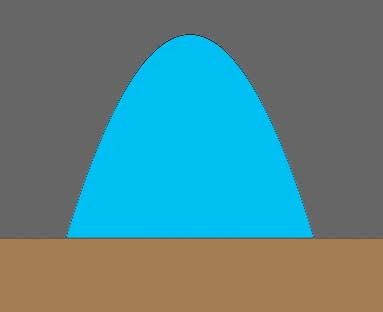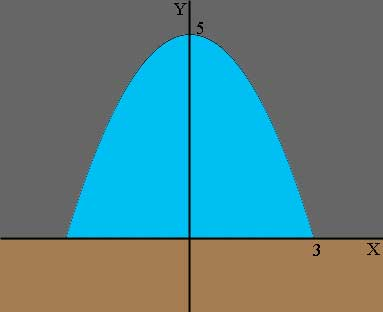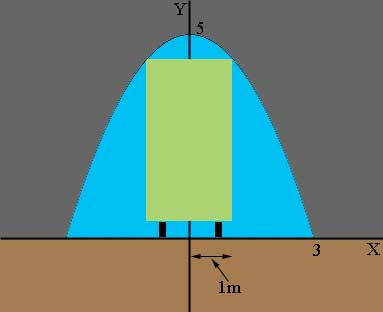SEARCH HOMEMath Central Quandaries & QueriesQuestion from Brennen, a student: A railway bridge over a road is in the shape of a parabola, and the bridge is 3 m high in the middle and 6 m wide at its base. A truck that is 2m wide is approaching the bridge. What is the maximum height that the truck can have and still pass under the bridge? Explain. Thank you!!Brennen,

A strange shape for a railway bridge. I would have said "A tunnel through a mountain is in the shape of a parabola ...".I put a coordinate system on the diagram with the X-axis on the road surface and the Y-axis passing through the vertex of the parabola.The parabola then has its equation of the form $y = a x^2 + b.$ From the diagram, when $x = 0, y = 5$ and when $x = 3, y = 0.$ Use these facts to solve for $a$ and $b.$ (Since the parabola opens downward $a$ will be negative.)

If the truck drives down the centre of the road then the side of the truck will be at $x = 1.$What is the height of the tunnel at $x = 1?$

PennyMath Central is supported by the University of Regina and The Pacific Institute for the Mathematical Sciences.